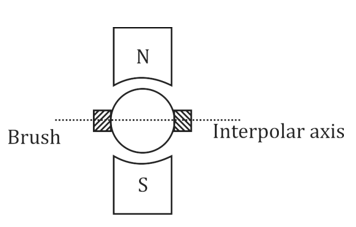Engineering Jobs   »   Electrical Engineering quizs   »   PSPCL Recruitment 2021, PSPCL-JE ELECTRICAL. PSPCL-JE...

# PSPCL-JE’21 EE: Daily Practices Quiz 05-Oct-2021

## PSPCL RECRUITMENT 2021

Punjab State Power Corporation Ltd. (PSPCL), a power generating and distributing company of the Government of Punjab state, has released the notification for recruitment to the post of Clerk, Revenue Accountant, Junior Engineer (Electrical), Assistant Lineman & Assistant Sub Station Attendant Posts .
A total of 75 vacancies have been announced for the recruitment process of JE ELECTRICAL Engineering.
Exam Dates: 10 N0V-21 to 17 Nov-21

## PSPCL-JE’21 EE: Daily Quiz

PSPCL-JE’21 EE: Daily Practices Quiz 05-Oct-2021

Each question carries 1 mark.
Negative marking: 1/4 mark
Total Questions: 06
Time: 08 min.

Q1. The condition for a maximum power output from dc motor is
(a) Eb=V
(b) Eb=V/2
(c) Eb=0
(d) Eb=V/√2

Q2. If the coefficient of coupling between two coils is increased, mutual inductance
between the coils
(a) Is decreased.
(b) Remains unchanged.
(c) Is increased.
(d) Changes depend on current only.

Q3. The brushes of a dc machine should be physically placed on the
(a) Armature in the polar axis.
(b) Armature in the interpolar axis.
(c) Commutator in the polar axis.
(d) Commutator in the interpolar axis.

Q4. A transformer is working at its full load and its efficiency is also maximum at which
iron los is 1000 watts. Then, its copper loss at half of full load will be:
(a) 250 watts
(b) 300 watts
(c) 400 watts
(d) 500 watts

Q5. A dynamometer type wattmeter responds to the
(a) Average value of active power
(b) Average value of reactive power
(c) Peak value of active power
(d) Peak value of reactive power

Q6. Which is the most severe fault?
(a) Phase to phase fault
(b) Phase to earth fault
(c) Three phases to ground fault
(d) Phase to phase to earth fault

## SOLUTIONS

S1. Ans.(b)
Sol. Maximum power developed in a DC machine.
For motor: Eb=V/2
For generator: V=E/2

S2. Ans.(c)
Sol. Coefficient of coupling:
K=M/√(L1.L2 )
For constant L1 and L2 if K is increased then M increases since M α K if L_1 and L_2 are
constants.

S3. Ans.(b)
Sol. Brushes of a dc machine should be physically placed on the armature in the interpolar axis as shown below.S4. Ans.(a)
Sol. The iron loss fat full load is 1000 Watts and maximum efficiency is obtained at full
So, x= √(Pi/Pcl )
∴ Full load copper loss = Ifl^2.R=1000 Watts
∴ Half load copper loss = (Ifl/2)^2×R
=1000/4=250 Watts

S5. Ans.(a)
Sol. A dynamometer gives average value of active power over a cycle.
P=Vrms Irms cosϕ

S6. Ans.(c)
Sol. LLLG or three – phase to ground fault is most severe and in this short-circuit current value is maximum.
Severity of fault:
LLLG > LLL > LLG > LL > LG

Sharing is caring!

Thank You, Your details have been submitted we will get back to you.